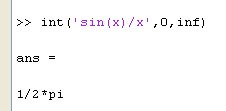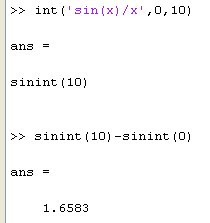## Friday, November 27, 2009

### SINE INTEGRAL IN SCIPY

THE SINE INTEGRAL

The Sine Integral is a very important function in Physics, Astronomy, Electrodynamics, Mathematical Physics,Optics and Signal Processing.A fundamental result in the sine integral is;This result is analytically proven using contour integrals concept from complex theory.

TRYING IT IN MATLABMATLAB gives excellent results, particularly for the special case of (0-inf )it gives correct value.

TRYING IT IN SCIPYIn Scipy the sine integral (and the cosine integral) is via (si,ci) = sici function. It yields excellent values for numbers, however for infinity it yields nan (not a number). This should probably be corrected with an exception in the sici module.

It is worth noting that for sufficiently high values (which tend to infinity) the desired result of 1.57.... ( = pi/2) is obtained, which confirms the numerical evaluation is correct

REFERENCES

(1)
sici
(2) sine integral